# choosability

## Choosability of Graph Powers ★★

Author(s): Noel

Question  (Noel, 2013)   Does there exist a function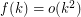such that for every graph,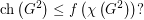## Bounding the on-line choice number in terms of the choice number ★★

Author(s): Zhu

Question   Are there graphs for whichis arbitrarily large?

## Choice number of complete multipartite graphs with parts of size 4 ★

Author(s):

Question   What is the choice number of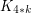for general?

## Choice Number of k-Chromatic Graphs of Bounded Order ★★

Author(s): Noel

Conjecture   Ifis a-chromatic graph on at mostvertices, then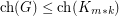.

## Circular choosability of planar graphs ★

Author(s): Mohar

Let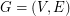be a graph. Ifandare two integers, a-colouring ofis a functionfrom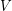tosuch thatfor each edge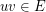. Given a list assignment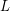of, i.e.~a mapping that assigns to every vertexa set of non-negative integers, an-colouring ofis a mappingsuch that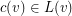for every. A list assignmentis a--list-assignment if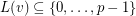andfor each vertex. Given such a list assignment, the graph G is--colourable if there exists a--colouring, i.e.is both a-colouring and an-colouring. For any real number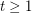, the graphis--choosable if it is--colourable for every--list-assignment. Last,is circularly-choosable if it is--choosable for any,. The circular choosability (or circular list chromatic number or circular choice number) of G is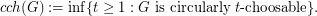Problem   What is the best upper bound on circular choosability for planar graphs?

Keywords: choosability; circular colouring; planar graphs

## Ohba's Conjecture ★★

Author(s): Ohba

Conjecture   If, then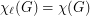.